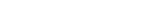MathExamle.com# Addition Subtraction Multiplication Division of integers

Create worksheets of mathematical examples with addition, subtraction, multiplication and division of integers, select type of a generator and viewing some samples of task

Creates examples with operations of addition, subtraction, multiplication and division of integers, the answers are always positive

3 Variants 3 Levels
 5240-10×16×24= 3110×30+5400÷27= 584×95-1280÷128= 9357-1200÷600×1219=
Variant: 2 Level: Hard

Creates examples with operations of multiplication and division of negative and positive integers

4 Variants 4 Levels
 (-20×2)÷(-4)= -350÷(-70÷14)= (6×60)÷(-40)= 36÷(-12÷2)=
Variant: 3 Level: Normal

Creates examples with operations of addition, subtraction, multiplication and division of integers, the answers can be negative

3 Variants 3 Levels
 72+36×2÷4= -33+6÷2= -17-(10+9)÷19= 6+(44-20)÷6=
Variant: A Level: Easy

Creates expressions with the operation of rounding integers to tens, hundreds or thousands

3 Variants 3 Levels
 304+2279≈ 4126≈ 6411≈ 9379-1047≈
Variant: A Level: Normal